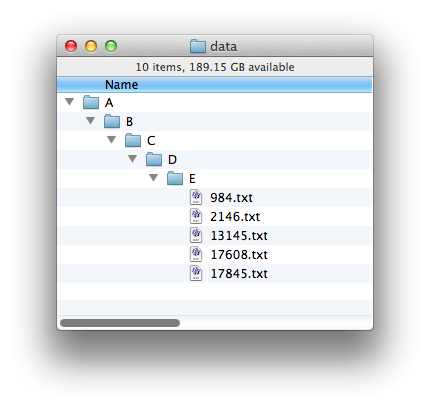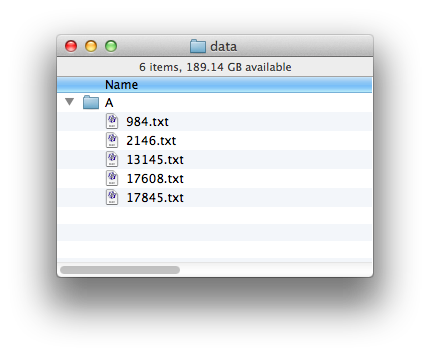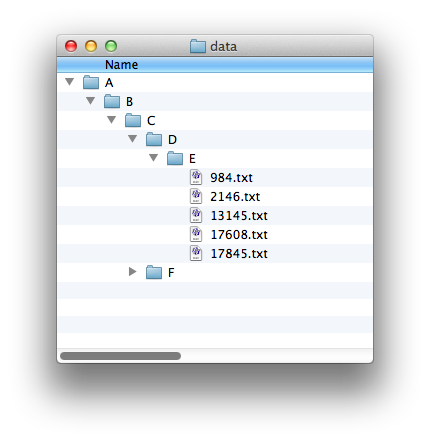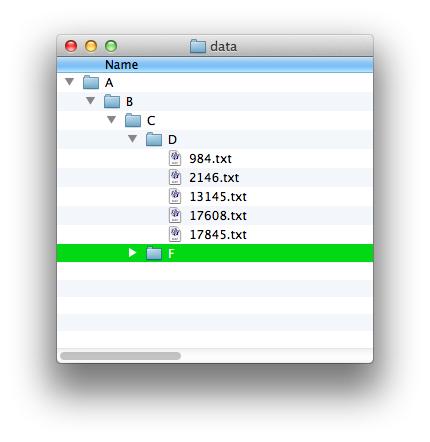## Learning Perl Challenge: Remove intermediate directoriesI often run into situations where I have directories that contain only one file, a subdirectory, with contain only one file, a subdirectory, and so on for a long chain, until I get to the interesting files. These situations come up when I have only part of a data set so the files that would be in other directories aren’t there, and I find it annoying to deal with these long directory specifications. So, this challenge is to fix that by collapsing those one-entry directories into a single one.

For example, you should take this structure, where you have A/B/C/D/E in a direct line with no other branches:and turn it into this one, with a single directory with the files that were at the end:However, you should only moves files up if the directory above it has only one entry (which must be a subdirectory!). In this example, A/B/C has two subdirectories in it:so the the files in E should only move up into D. Otherwise, the files from the two branches in C would get mixed up with each other.Leave a comment

## 5 Comments.

1.Szymon Sokół

In the second example, shouldn’t D and F end up in A, since B contains *only* C?

•brian d foy

I hadn’t thought about that. I’ll let you decide!

2.abbypan
```#!/usr/bin/perl
use strict;
use warnings;
use File::Path qw(remove_tree);
use File::Copy qw(move);

my (\$dir) = @ARGV;
short_dir(\$dir) ;

sub short_dir {
my (\$start, \$now) = @_;
return unless(-d \$start);
\$now ||= \$start;

my @subnodes = glob("\$now/*");
my @subdirs = grep { -d \$_ } @subnodes;

if(\$#subdirs>0){
short_dir(\$_) for @subdirs;
}elsif(\$#subdirs==0){
short_dir(\$start, \$subdirs);
}else{
return if(\$start eq \$now);
move(\$_, "\$start/") for @subnodes;
my @dirs = grep { -d \$_ } glob("\$start/*");
remove_tree(\$dirs);
}
}
```
3.E. Choroba

Not as easy as it seems. My solution works as Szymon proposed. Some details would still need discussion with the client, though 🙂 For example, each empty directory without siblings disappears. The script probably does not work correctly for /, but I did not have enough courage to test it. Also, I might have used a bit more than Learning Perl teaches – I have read more books.

```#!/usr/bin/perl
use warnings;
use strict;

use Cwd 'abs_path';
use constant SELF_OR_PARENT => qr/^\.\.?\$/;

sub collapse {
my \$dir = shift;
opendir my(\$DH), \$dir;
my \$count = 0;
my \$previous;
DIR:
while (my \$file = readdir \$DH) {
next DIR if \$file =~ SELF_OR_PARENT;
last DIR if ++\$count > 1;
\$previous = \$file;
}

if (\$count == 1
and defined \$previous
and -d (my \$fullpath = "\$dir/\$previous")
) {
my (\$path, \$dirname) = \$dir =~ m=(.*)/(.*)=;
rename \$fullpath, "\$dir/../_\$dirname" or die \$!;
rmdir \$dir or die \$!;
rename "\$path/_\$dirname", \$dir or die \$!;
collapse(\$dir);

} else {
opendir my (\$DH), \$dir;
while (my \$subdir = readdir \$DH) {
collapse("\$dir/\$subdir") if -d "\$dir/\$subdir"
and \$subdir !~ SELF_OR_PARENT;
}
}
}

my \$top = shift;
die "\$top is not a dir" unless -d \$top;
\$top = abs_path(\$top);

collapse(\$top);
```
4.P. Vlad

I’m mainly a C++ programmer, but I tried my hand at perl this time. I used a recursive function and shell commands.

```#!/usr/bin/perl

sub checkAndDelete{
if(!(-d \$_)){
return;
}
my \$command="ls ".\$_;
\$scc=`\$command`;
\$scc=~s/ /\n/;
my @files=split("\n",\$scc);
my [email protected];

my \$fprefix=\$_;
my \$lastc=substr(\$fprefix,-1);
if(!(\$lastc eq "/")){
\$fprefix=\$fprefix."/";
}
if(\$size==1 and -d((\$fprefix.\$files))){
my \$cpcom="cp -a ".\$fprefix.\$files."/. ".\$fprefix;
print(\$cpcom."\n");
system(\$cpcom);
system("rm -r ".\$fprefix.\$files);
}
@files=split("\n",(`\$command`));
[email protected];

for(my \$i=0;\$i<\$size;\$i++){
&checkAndDelete((\$fprefix.\$files[\$i]));
}
}

@ARGV>0 or die 'Insufficient arguments';
-d \$ARGV or die 'Incorrect path';

&checkAndDelete(\$ARGV);
```